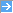ASP本类阅读TOP10 ・asp常用数据库连接方法和技巧・无组件生成BMP验证码・一些常用的辅助代码 (网络收藏)・JavaScript实现的数据表格：冻结列、调整列宽和客户端排序・VisualStudio.NET_2003及其 MSDN 下载地址・ASP模拟MVC模式编程・图片以二进制流输出到网页・MD5加密算法 ASP版・ASP.NET编程中的十大技巧・改进 ASP 的字符串处理性能

 可以把文章标题中的英文单词的首字母变成大写的函数 作者：未知 来源：月光软件站 加入时间：2005-2-28　月光软件站 功能说明: 可以把文章标题中的英文单词的首字母变成大写:) 效果演示:<% function PCase(strInput)     'Variable declaration.     Dim strArr     Dim tmpWord     Dim tmpString     Dim last               'Create an array To store Each word In the String separately.     strArr = split(strInput," ")               if ubound(strArr) > 0 Then      For x = lbound(strArr) To ubound(strArr)       'Set Each word To lower Case initially.       strArr(x) = LCase(strArr(x))                   'Skip the unimportant words.       Select Case strArr(x)        Case "a"        Case "an"        Case "and"        Case "but"        Case "by"        Case "for"        Case "in"        Case "into"        Case "is"        Case "of"        Case "off"        Case "on"        Case "onto"        Case "or"        Case "the"        Case "to"        Case "a.m."         strArr(x) = "A.M."        Case "p.m."         strArr(x) = "P.M."        Case "b.c."         strArr(x) = "B.C."        Case "a.d."         strArr(x) = "A.D."        Case Else                 'Capitalize the first letter, but don't forget To take into account that         'the String may be in Single or Double quotes.         if len(strArr(x)) > 1 Then          if mid(strArr(x),1,1) = "'" or mid(strArr(x),1,1) = """" Then           tmpWord = mid(strArr(x),1,1) & Ucase(mid(strArr(x),2,1)) & mid(strArr(x),3,len(strArr(x))-2)          Else           tmpWord = Ucase(mid(strArr(x),1,1)) & mid(strArr(x),2,len(strArr(x))-1)          End if          strArr(x) = tmpWord         End if                End Select                     'The unimportant words may need To be capitalized if they follow a dash, colon,       'semi-colon, Single quote or Double quote.       if x > 0 Then        if instr(strArr(x-1),"-") _        or instr(strArr(x-1),":") _        or instr(strArr(x-1),";") Then         tmpWord = Ucase(mid(strArr(x),1,1)) & mid(strArr(x),2,len(strArr(x))-1)         strArr(x) = tmpWord        End if       End if             Next     Else      strArr(0) = LCase(strArr(0))     End if               'Make sure the first word In the array is upper case, but don't forget To take into account     'that the String may be in Single or Double quotes.     if mid(strArr(0),1,1) = "'" or mid(strArr(0),1,1) = """" Then      tmpWord = mid(strArr(0),1,1) & Ucase(mid(strArr(0),2,1)) & mid(strArr(0),3,len(strArr(0))-2)     Else      tmpWord = Ucase(mid(strArr(0),1,1)) & mid(strArr(0),2,len(strArr(0))-1)     End if     strArr(0) = tmpWord               'Also, make sure the last word In the array is upper case, but don't forget To take into account     'that the String may be in Single or Double quotes.     last = ubound(strArr)     if mid(strArr(last),1,1) = "'" or mid(strArr(last),1,1) = """" Then      tmpWord = mid(strArr(last),1,1) & Ucase(mid(strArr(last),2,1)) & mid(strArr(0),3,len(strArr(last))-2)     Else      tmpWord = Ucase(mid(strArr(last),1,1)) & mid(strArr(last),2,len(strArr(last))-1)     End if     strArr(last) = tmpWord               'Rebuild the whole String from the array parts.     For x = lbound(strArr) To ubound(strArr)      tmpString = tmpString & strArr(x) & " "     Next          PCase = tmpString    End function%>相关文章：相关软件：